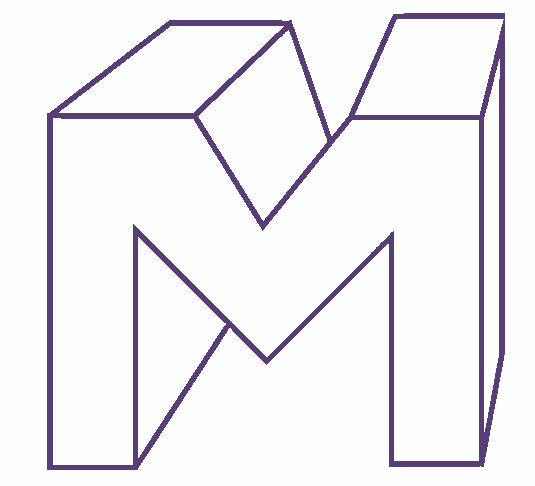Find the number of faces in the given shape.

# Find the number of faces in the given shape.1. A
14
2. B
20
3. C
16
4. D
10

Fill Out the Form for Expert Academic Guidance!l

+91

Live ClassesBooksTest SeriesSelf Learning

Verify OTP Code (required)

### Solution:

The given shape isWe know that each flat portion of a polyhedron is referred to as its face.
Therefore, the number of faces in the given shape = 14.
The correct option is (1).

## Related content

 Important Topic of Physics: Reynolds Number Distance Speed Time Formula Refractive Index Formula Mass Formula Electric Current Formula Electric Power Formula Resistivity Formula Weight Formula Linear Momentum Formula Non Contact Force+91

Live ClassesBooksTest SeriesSelf Learning

Verify OTP Code (required)